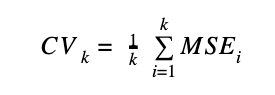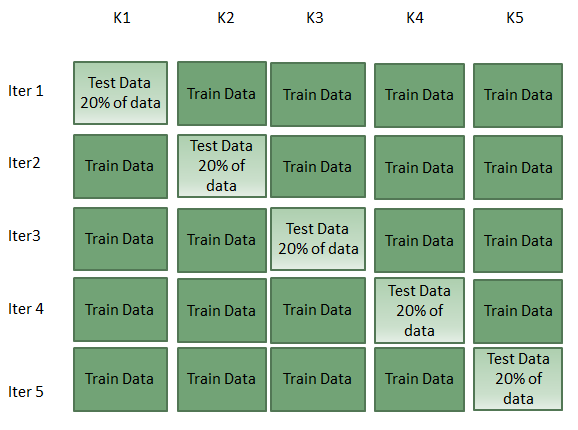# Cross Validation to Avoid Overfitting in Machine Learning

Cross validation is a technique used to determine how the results of a machine learning model could be generalized to new, unseen data. The training error associated with a model might underestimate the test error of the model, so the Cross Validation approach provides a mechanism to get the MSE test with the current dataset without the need of finding new data to test the model.

Basically, to perform Cross Validation we need to keep aside a portion of the data that is not used to train the model.  The goal of Cross Validation is to estimate the test error of the model, by holding a subset of the dataset in order to use them as test observations. This approach gives a more accurately estimate of the test error.

### Validation Set Approach

The classical method for training and testing a dataset is called the Validation Set approach. We have used this approach in both examples of Multivariate linear regression and for the Classifier Forecasting.  This consists of splitting the dataset into a train and a test set. Commonly around 80% of the data is used to train the dataset and the other 20 % of the data is used as the test set.

The splitting is done in chronological order, where the first two thirds represent the first two thirds of the historical data. One of the drawbacks of this method is that by choosing different length for the train and the test data, the model performance can vary significantly.

Likewise, if we have a limited amount of data, there is a possibility of high bias because we would miss some information about the data that was not used for training. If the amount of data is huge and the train and test data have the same distribution, this approach is acceptable.

The second approach to address overfitting is to train and test the model using the method called K-Fold Cross Validation.

### K-Fold Cross Validation

K-Fold Cross Validation is a more sophisticated approach that generally results in a less biased model compared to other methods. This method consists in the following steps:

1. Divides the n observations of the dataset into k mutually exclusive and equal or close-to-equal sized subsets known as “folds”.
2. Fit the model using k-1 folds as the training set and one fold (kth) as the test set. After each iteration has been finished, store the error of the model.
3. Repeat this process k times using one different fold every time as a test set and the remaining folds (k-1) as the training set.
4. Once all the iterations have finished, take the mean of the k models. This would be the Mean Squared Error of the model.

The error model in using the K-Fold cross validation has the following formula:Error Model Formula

An important consideration of this approach is the selection of the number of folds. The choice of the number of folds should be done on the basis that each fold needs to have enough data points to provide a fair estimate of the model performance. On the other hand, the k number should not be so small such as 2, in order to have enough trained models to assess the model performance.

K-Fold Cross Validation method